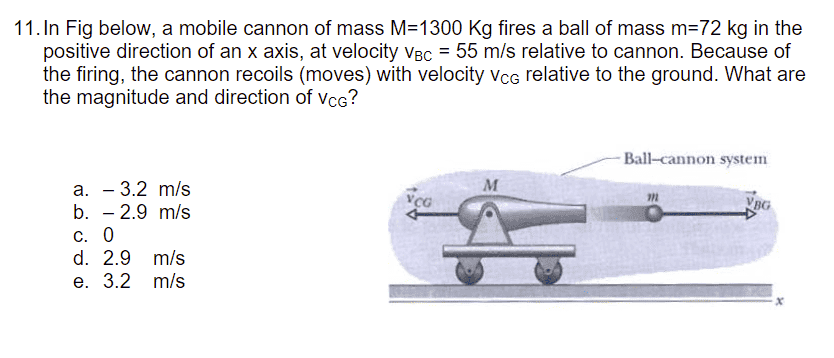# Simple Canon Question (Conservation Of Momentum): Frame of Reference

Kermit_the_Phrog
Homework Statement:
In Fig below, a mobile cannon of mass M=1300 Kg fires a ball of mass m=72 kg in the positive direction of an x axis, at velocity Vbc= 55 m/s relative to cannon. Because of the firing, the cannon recoils (moves) with velocity Vcg relative to the ground. What are the magnitude and direction of Vcg?
Relevant Equations:
Pi=PfSince Pi = Pf,

0 = MbVbg + McVcg

I just need to express Vbg in terms of Vbc and Vcg (that is, I need to express the velocity of the ball relative to the ground in terms that I know/want to solve for):

by reference frames:

Vbc = Vbg + Vcg
so Vbg = Vbc -Vcg

Now I can sub in and solve

0 = MbVbg + McVcg
0 = Mb(Vbc -Vcg) + McVcg
0 = MbVbc - MbVcg +McVcg
MbVcg - McVcg = MbVbc
Vcg(Mb - Mc) = MbVbc

therefore,
Vcg = 72*55/(72-1300)
= -3.2 m/s
which would be a.

HOWEVER the teacher's answer key states that the answer is b, -2.9 m/s.
this answer is obtained if the denominator was " (- mb - mc) ".

Thus, did I just mess up somewhere with my signs? I can't find mistake, and any help deducing this would be appreciated, as I was pretty confident about my answer until seeing that it was wrong.

Homework Helper
Gold Member
Thus, did I just mess up somewhere with my signs?

Yes. The signs are inconsistent between the two equations

0 = MbVbg + McVcg
Vbg = Vbc -Vcg

Give it some more thought.

•Kermit_the_Phrog
Kermit_the_Phrog
Yes. The signs are inconsistent in the two equations

0 = MbVbg + McVcg
Vbg = Vbc -Vcg

Give it some more thought.

Is the momentum equation supposed to be

0 = MbVbg - McVcg

Because Vcg is pointed in the opposite direction to Vbg?

Kermit_the_Phrog
Give it some more thought.

If that's true, you get

Vcg = MbVbc/(Mb+Mc)
= 72*55/(72+1300)
= 2.9

which is then negative through intuition (since its moving in the opposite direction?)

Though i suppose this works, I feel like there's a better / more accurate way to show the signs in my algebra.

Thoughts?

Homework Helper
Gold Member
2022 Award
Is the momentum equation supposed to be

0 = MbVbg - McVcg

Because Vcg is pointed in the opposite direction to Vbg?
Assuming you are taking right as positive in all cases, your original momentum equation was correct.
In writing relative motion equations, the safest approach is always to write them in the form
Motion of A relative to B = Motion of A relative to common frame - Motion of B relative to common frame.

•Kermit_the_Phrog
Kermit_the_Phrog
Assuming you are taking right as positive in all cases, your original momentum equation was correct.
In writing relative motion equations, the safest approach is always to write them in the form
Motion of A relative to B = Motion of A relative to common frame - Motion of B relative to common frame.

Ah so if its the other equation, I was wrong initially

so at first it would be:
Vbc = Vbg - Vcg

meaning
Vbg = Vbc + Vcg

Then the rest is the same:

0 = MbVbg + McVcg
0 = Mb(Vbc + Vcg) +McVcg
0 = MbVbc + MbVcg +McVcg
Vcg = -MbVbc/(Mb+Mc)
= -72*55/(72+1300)
= -2.9m/s

And THATS correct

So it was just a dumb mistake with the first reference frame equation

•